#Function Repository Resource:

# RunOnQuit

Set an evaluation to run when the Wolfram Language kernel session is terminated

Contributed by: Richard Hennigan (Wolfram Research)
 ResourceFunction["RunOnQuit"][eval] adds eval to a list of evaluations to complete when the current kernel quits.

## Details and Options

Each evaluation of ResourceFunction["RunOnQuit"][expr] prepends expr to the list of evaluations to run at kernel exit.
ResourceFunction["RunOnQuit"] does not interfere with existing code defined for \$Epilog.

## Examples

### Basic Examples

Evaluate an expression when the Wolfram Language kernel session is terminated:

 In:=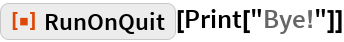Out=In:=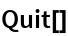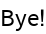In:=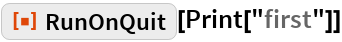Out=In:=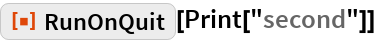Out=In:=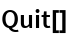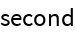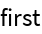### Scope

RunOnQuit[expr] is similar to \$Epilog := expr, but the file end.m is still read (if available):

 In:=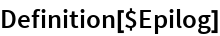Out=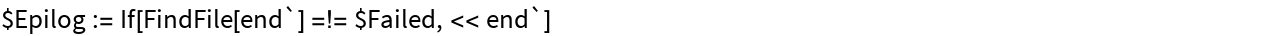In:=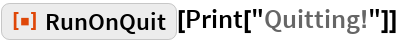Out=In:=Out=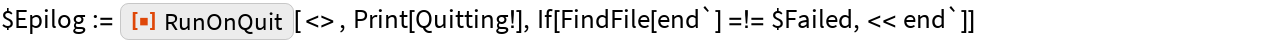Each time RunOnQuit is used, code is prepended to the sequence of evaluations in \$Epilog:

 In:=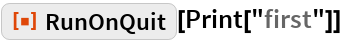Out=In:=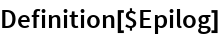Out=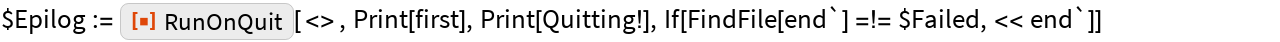In:=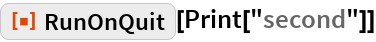Out=In:=Out=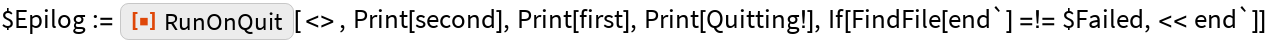In:=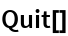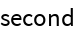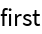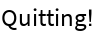If \$Epilog is undefined, RunOnQuit[expr] defines it:

 In:=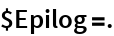In:=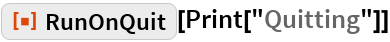Out=In:=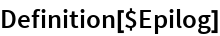Out=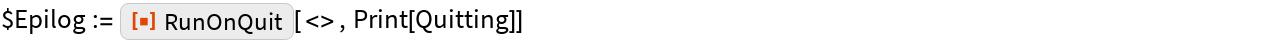Evaluations will then be prepended as normal:

 In:=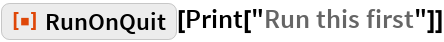Out=In:=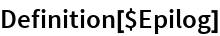Out=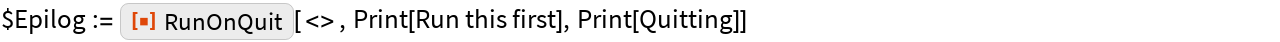In:=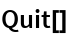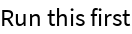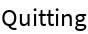### Applications

Create a notebook that only persists for the current kernel session:

 In:=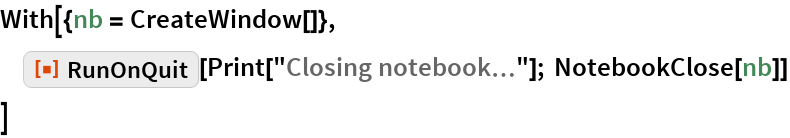Out=Make sure you are logged out of the cloud when the kernel quits:

 In:=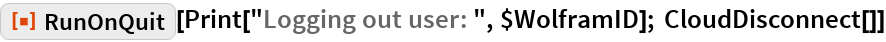Out=Clean up any messes that you make:

 In:=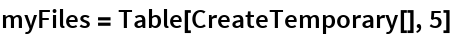Out=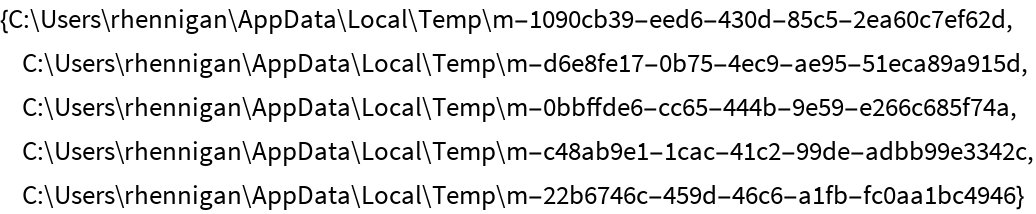In:=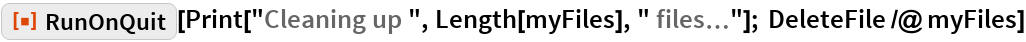Out=Run all evaluations on quit:

 In:=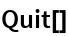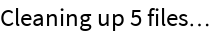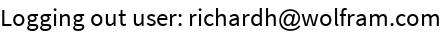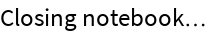### Possible Issues

\$Epilog will not be evaluated from subkernels:

 In:=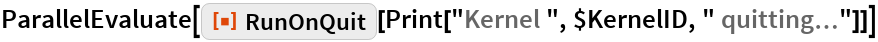Out=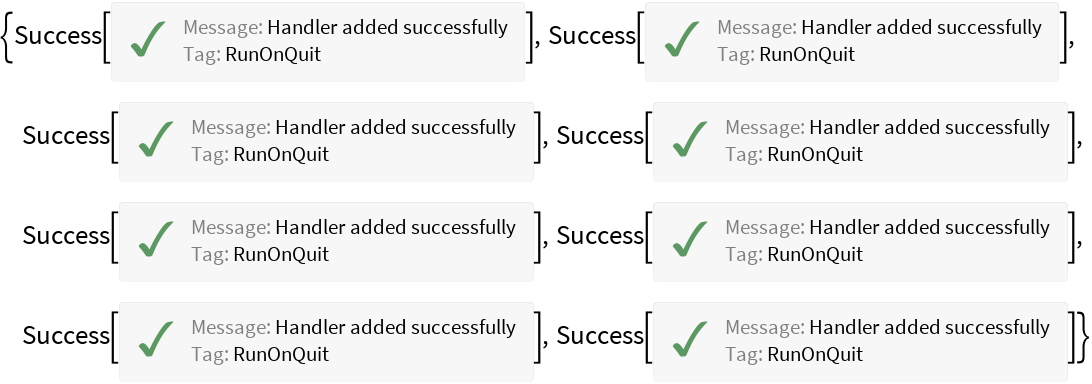In:=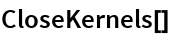Out=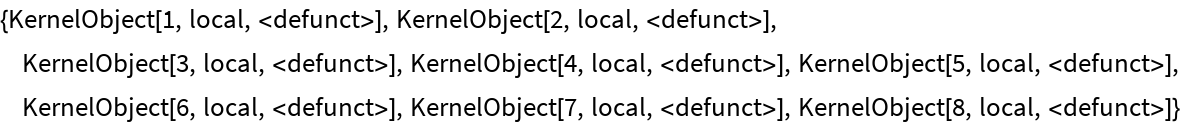RunOnQuit will fail if \$Epilog is Protected:

 In:=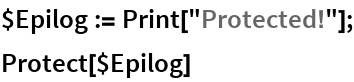Out=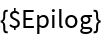In:=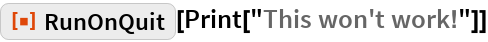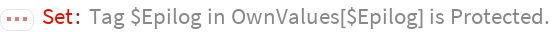Out=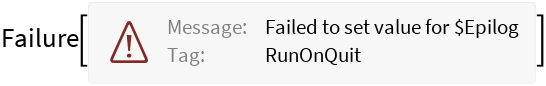In:=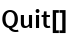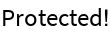## Requirements

Wolfram Language 11.3 (March 2018) or above